Testking offers free demo for 98-381 exam. "Introduction to Programming Using Python", also known as 98-381 exam, is a Microsoft Certification. This set of posts, Passing the Microsoft 98-381 exam, will help you answer those questions. The 98-381 Questions & Answers covers all the knowledge points of the real exam. 100% real Microsoft 98-381 exams and revised by experts!

NEW QUESTION 1
HOTSPOT
You are developing a Python application for your company. You write the following code: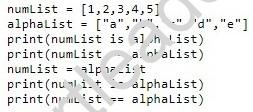Use the drop-down menus to select the answer choice that answers each question based on the information presented in the code segment.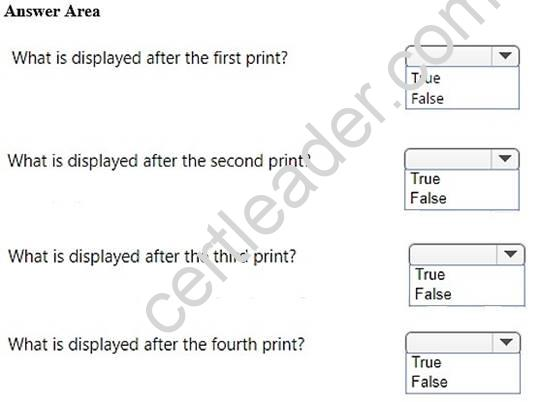• A. Mastered
• B. Not Mastered

Explanation:
References: https://www.w3resource.com/python/python-list.php

NEW QUESTION 2
You develop a Python application for your school.
You need to read and write data to a text file. If the file does not exist, it must be created. If the file has content, the content must be removed.
Which code should you use?

• A. open(“local_data”, “r”)
• B. open(“local_data”, “r+”)
• C. open(“local_data”, “w+”)
• D. open(“local_data”, “w”)

Explanation:
References: https://pythontips.com/2014/01/15/the-open-function-explained/

NEW QUESTION 3
DRAG DROP
You are writing a Python program. The program collects customer data and stores it in a database.
The program handles a wide variety of data.
You need to ensure that the program handles the data correctly so that it can be stored in the database correctly.
Match the data type to the code segment. To answer, drag the appropriate data type from the column on the left to its code segment on the right. Each data type may be used once, more than once, or not at all.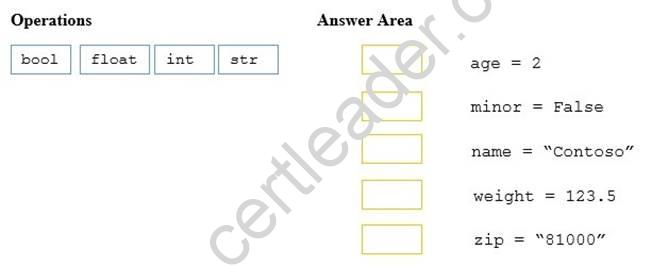• A. Mastered
• B. Not Mastered

Explanation:
References: https://www.w3resource.com/python/python-data-type.php

NEW QUESTION 4
You are creating a function that manipulates a number. The function has the following requirements:
✑ A float is passed into the function
✑ The function must take the absolute value of the float
✑ Any decimal points after the integer must be removed
Which two math functions should you use? Each correct answer is part of the solution? (Choose two.)

• A. math.fmod(x)
• B. math.frexp(x)
• C. math.floor(x)
• D. math.ceil(x)
• E. math.fabs(x)

Explanation:
References: https://docs.python.org/2/library/math.html#number-theoretic-and- representation-functions

NEW QUESTION 5
HOTSPOT
During school holidays, you volunteer to explain some basic programming concepts to younger siblings.
You want to introduce the concept of data types in Python. You create the following three code segments: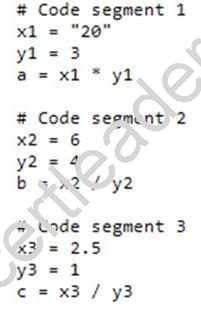You need to evaluate the code segments.
For each of the following statements, select Yes if the statement is true. Otherwise, select No.
NOTE: Each correct selection is worth one point.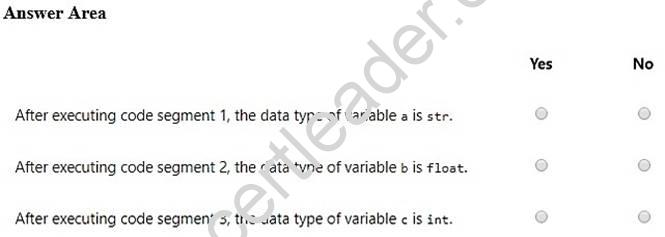• A. Mastered
• B. Not Mastered

Explanation:
References: https://www.w3resource.com/python/python-data-type.php

NEW QUESTION 6
You are writing code that generates a random integer with a minimum value of 5 and a maximum value of 11.
Which two functions should you use? Each correct answer presents a complete solution. (Choose two.)

• A. random.randint(5, 12)
• B. random.randint(5, 11)
• C. random.randrange(5, 12, 1)
• D. random.randrange(5, 11, 1)

Explanation:
References: https://docs.python.org/3/library/random.html#

NEW QUESTION 7
The ABC company is creating a program that allows customers to log the number of miles biked. The program will send messages based on how many miles the customer logs.
You create the following Python code. Line numbers are included for reference only.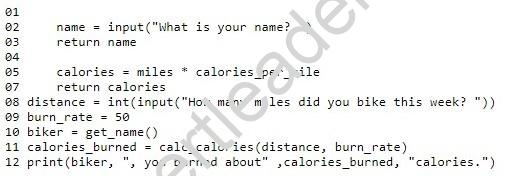You need to define the two required functions.
Which code segments should you use for line 01 and line 04? Each correct answer presents part of the solution? (Choose two.)

• A. 01 def get_name():
• B. 01 def get_name(biker):
• C. 01 def get_name(name):
• D. 04 def calc_calories():
• E. 04 def calc_calories(miles, burn_rate):
• F. 04 def calc_calories(miles, calories_per_mile):

Explanation:
References: https://www.w3resource.com/python/python-user-defined-functions.php

NEW QUESTION 8
HOTSPOT
You are coding a math utility by using Python. You are writing a function to compute roots.
The function must meet the following requirements: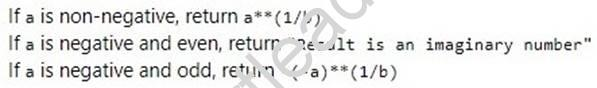How should you complete the code? To answer, select the appropriate code segments in the answer area.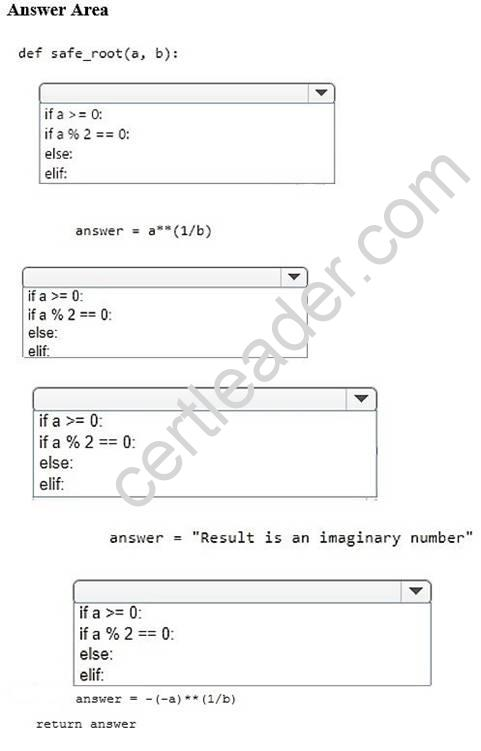• A. Mastered
• B. Not Mastered

Explanation:
References: https://www.w3resource.com/python/python-if-else-statements.php

NEW QUESTION 9
HOTSPOT
You create the following program to locate a conference room and display the room name. Line numbers are included for reference only.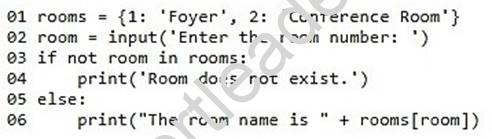Colleagues report that the program sometimes produces incorrect results.
You need to troubleshoot the program. Use the drop-down menus to select the answer choice that answers each question based on the information presented in the code segment.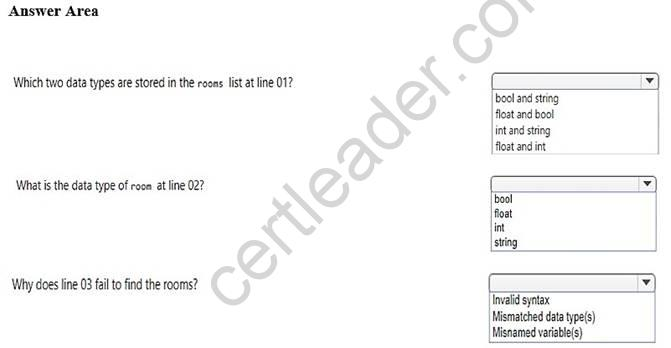• A. Mastered
• B. Not Mastered

Explanation:
References:
https://www.w3resource.com/python/python-data-type.php https://www.w3resource.com/python/python-if-else-statements.php

NEW QUESTION 10
Evaluate the following Python arithmetic expression: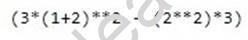What is the result?

• A. 3
• B. 13
• C. 15
• D. 69

Explanation:
References: http://www.mathcs.emory.edu/~valerie/courses/fall10/155/resources/op_precedence.html

NEW QUESTION 11
HOTSPOT
For each of the following statements, select Yes if the statement is true. Otherwise, select No.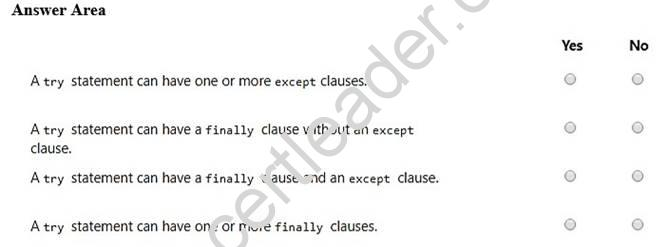• A. Mastered
• B. Not Mastered

Explanation:
References: https://docs.python.org/2.0/ref/try.html

NEW QUESTION 12
HOTSPOT
The ABC company is building a basketball court for its employees to improve company morale.
You are creating a Python program that employees can use to keep track of their average score.
The program must allow users to enter their name and current scores. The program will output the user name and the user’s average score. The output must meet the following requirements:
✑ The user name must be left-aligned.
✑ If the user name has fewer than 20 characters, additional space must be added to the right.
✑ The average score must have three places to the left of the decimal point and one place to the right of the decimal (XXX.X).
How should you complete the code? To answer, select the appropriate code segments in the answer area.
NOTE: Each correct selection is worth one point.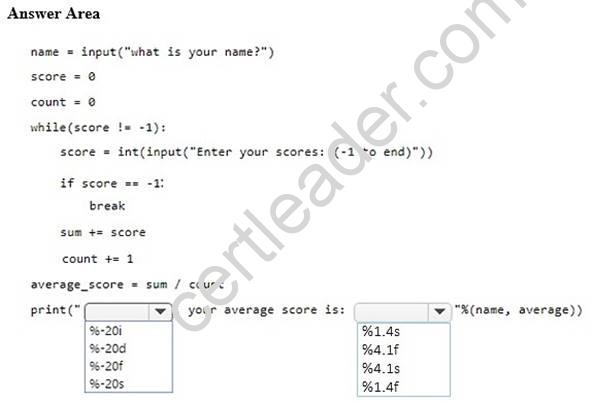• A. Mastered
• B. Not Mastered

Explanation:
References: https://www.python-course.eu/python3_formatted_output.php

NEW QUESTION 13
HOTSPOT
You create a function to calculate the power of a number by using Python. You need to ensure that the function is documented with comments.
You create the following code. Line numbers are included for reference only.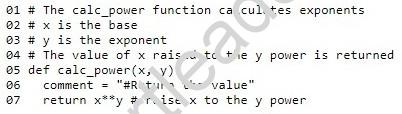For each of the following statements, select Yes if the statement is true. Otherwise, select No.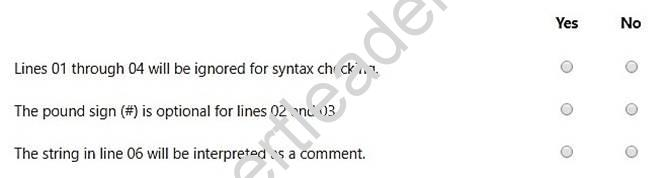• A. Mastered
• B. Not Mastered

Explanation:

NEW QUESTION 14
HOTSPOT
You are developing a Python application for an online product distribution company.
You need the program to iterate through a list of products and escape when a target product ID is found.
How should you complete the code? To answer, select the appropriate code segments in the answer area.
NOTE: Each correct selection is worth one point.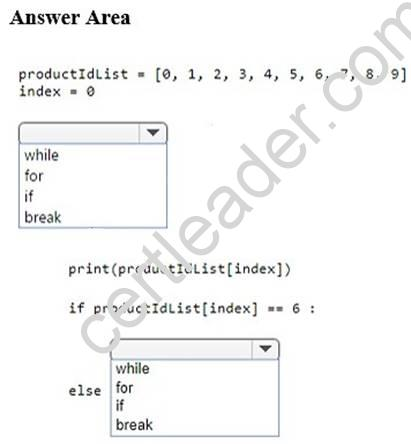• A. Mastered
• B. Not Mastered

Explanation:
References: https://www.w3resource.com/python/python-while-loop.php

NEW QUESTION 15
HOTSPOT
The ABC Video company needs a way to determine the cost that a customer will pay for renting a DVD. The cost is dependent on the time of day the DVD is returned. However, there are also special rates on Thursdays and Sundays. The fee structure is shown in the following list:
✑ The cost is \$1.59 per night.
✑ If the DVD is returned after 8 PM, the customer will be charged an extra day.
✑ If the video is rented on a Sunday, the customer gets 30% off for as long as they keep the video.
✑ If the video is rented on a Thursday, the customer gets 50% off for as long as they keep the video.
You need to write code to meet the requirements.
How should you complete the code? To answer, select the appropriate code segments in the answer area.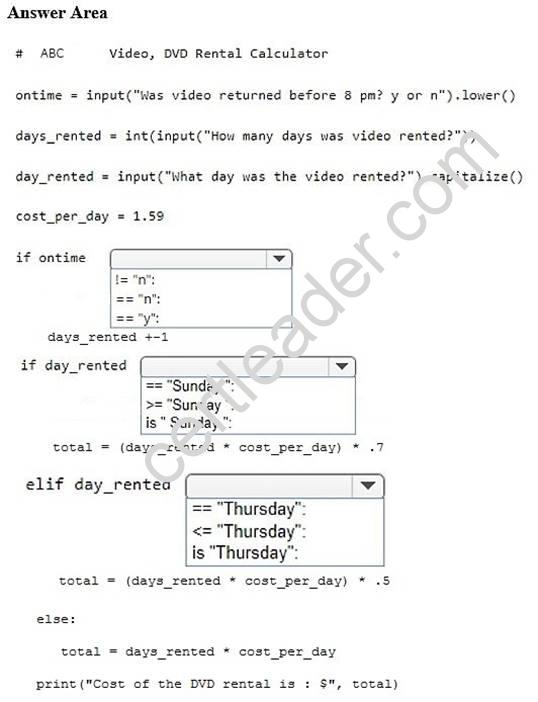• A. Mastered
• B. Not Mastered

Explanation:
References:
https://www.w3resource.com/python/python-operators.php https://www.w3resource.com/python/python-if-else-statements.php

NEW QUESTION 16
HOTSPOT
You find errors while evaluating the following code. Line numbers are included for reference only.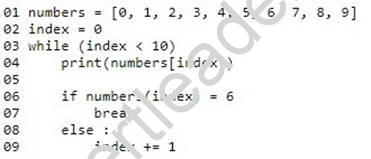You need to correct the code at line 03 and line 06.
How should you correct the code? Use the drop-down menus to select the answer choice
that answers each question based on the information presented in the code segment. NOTE: Each correct selection is worth one point.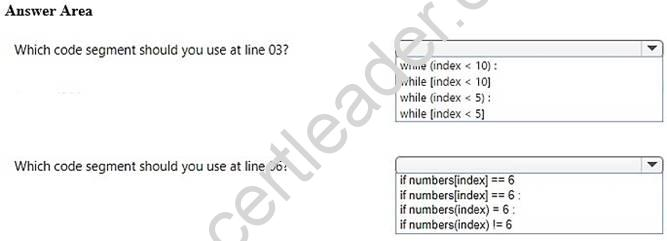• A. Mastered
• B. Not Mastered

Explanation:
References: https://www.w3resource.com/python/python-while-loop.php

NEW QUESTION 17
You are writing an application that uses the sqrt function. The program must reference the function using the name squareRoot.
You need to import the function. Which code segment should you use?

• A. import math.sqrt as squareRoot
• B. import sqrt from math as squareRoot
• C. from math import sqrt as squareRoot
• D. from math.sqrt as squareRoot

Explanation:
References: https://infohost.nmt.edu/tcc/help/pubs/python/web/import-statement.html

NEW QUESTION 18
DRAG DROP
You are writing a function that works with files.
You need to ensure that the function returns None if the file does not exist. If the file does exist, the function must return the first line.
You write the following code: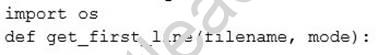In which order should you arrange the code segments to complete the function? To
answer, move all code segments from the list of code segments to the answer area and arrange them in the correct order.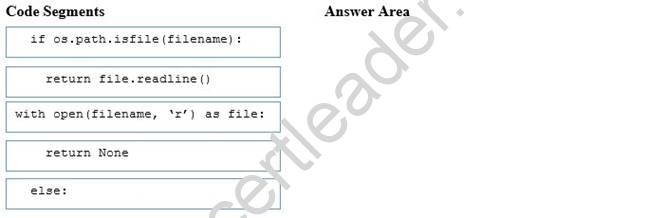• A. Mastered
• B. Not Mastered

Explanation:
References: http://effbot.org/zone/python-with-statement.htm

NEW QUESTION 19
This question requires that you evaluate the underlined text to determine if it is correct.
You write the following code: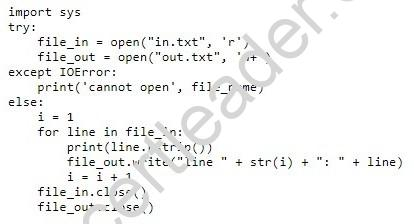The out.txt file does not exist. You run the code. The code will execute without error. Review the underlined text. If it makes the statement correct, select “No change is needed”.
If the statement is incorrect, select the answer choice that makes the statement correct.

• A. No change is needed
• B. The code runs, but generates a logic error
• C. The code will generate a runtime error
• D. The code will generate a syntax error